# Class 8 Maths Chapter 2 Exercise 2.5 Solutions

In this page we have Class 8 Maths Chapter 2 Exercise 2.5 Solutions.This exercise on question on equation which can be reduced to form of linear equation Hope you like them and do not forget to like , social share and comment at the end of the page.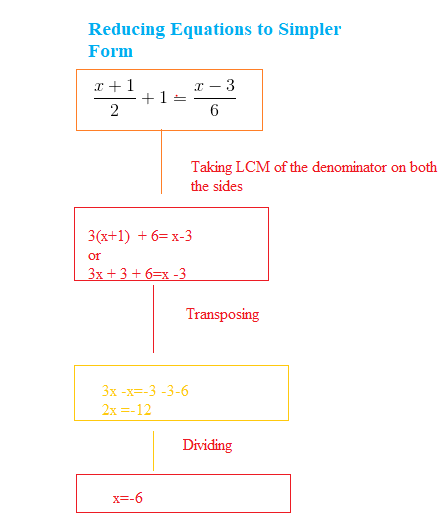## Class 8 Maths Chapter 2 Exercise 2.5 Solutions

Question 1
Solve the linear equation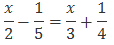L.C.M. of the denominators, 2, 3, 4, and 5, is 60.
So Multiplying both sides by 60, we obtain
$30x- 12 = 20x+ 15$
Transposing 20x to R.H.S and 12 to L.H.S, we obtain
$30x- 20x= 15 + 12$
10x= 27
X=27/10=2.7

Question 2
Solve the linear equation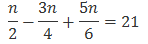L.C.M. of the denominators, 2, 4, and 6, is 12.
Multiplying both sides by 12, we obtain
$6n- 9n+ 10n= 252$
7n= 252
Dividing by 7 on both the sides
n=36

Question 3
Solve the linear equation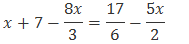L.C.M. of the denominators, 2, 3, and 6, is 6.
Multiplying both sides by 6, we obtain
6x+ 42 - 16x= 17 - 15x
Transposing 15x to RHS and 42 to LHS
6x- 16x+ 15x= 17 - 42
5x= -25
Dividing by 5 on both the sides
x=-5

Question 4
Solve the linear equation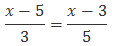L.C.M. of the denominators, 3 and 5, is 15.
Multiplying both sides by 15, we obtain
$5(x- 5) = 3(x- 3)$
$5x- 25 = 3x- 9$
Transposing 3x to RHS and 25 to LHS
5x- 3x= 25 - 9
2x= 16
Dividing both the sides by 2
x=8

Question 5
Solve the linear equation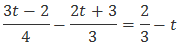L.C.M. of the denominators, 3 and 4, is 12.
Multiplying both sides by 12, we obtain
$3(3t- 2) - 4(2t+ 3) = 8 - 12t$
$9t- 6 - 8t- 12 = 8 - 12t$
Transposing 12t to RHS and 6 and 12 to LHS
9t- 8t+ 12t= 8 + 6 + 12
13t= 26
Dividing by 13 on both the sides
t=2

Question 6
Solve the linear equation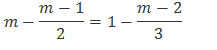L.C.M. of the denominators, 2 and 3, is 6.
Multiplying both sides by 6, we obtain
$6m- 3(m- 1) = 6 - 2(m- 2)$
$6m- 3m+ 3 = 6 - 2m+ 4$
Transposing 2m to RHS and 3 to LHS
6m- 3m+ 2m= 6 + 4 - 3
5m= 7
Dividing by 5 on both the sides
m=7/5

Question 7
Simplify and solve the linear equation $3(t- 3) = 5(2t+ 1)$

$3(t- 3) = 5(2t+ 1)$
3t- 9 = 10t+ 5
-9 - 5 = 10t- 3t
-14 = 7t
t=-2
Question 8
Simplify and solve the linear equation $15(y- 4) - 2(y- 9) + 5(y+ 6) = 0$
$15(y- 4) - 2(y- 9) + 5(y+ 6) = 0$
15y- 60 - 2y+ 18 + 5y+ 30 = 0
18y- 12 = 0
18y= 12 y=12/18=2/3
Question 9
Simplify and solve the linear equation
$3(5z- 7) - 2(9z- 11) = 4(8z- 13)-17$
$3(5z- 7) - 2(9z- 11) = 4(8z- 13)-17$
15z- 21 - 18z+ 22 = 32z- 52 - 17
-3z+ 1 = 32z- 69
-3z- 32z= -69 - 1
-35z= -70
z=2

Question 10
Simplify and solve the linear equation $0.25(4f- 3) = 0.05(10f- 9)$
$0.25(4f- 3) = 0.05(10f- 9)$
Multiplying both sides by 20, we obtain
$5(4f- 3) = 10f- 9$
20f- 15 = 10f- 9
20f- 10f= - 9 + 15
10f= 6
f==6/10=3/5

## Summary

1. Class 8 Maths Chapter 2 Exercise 2.5 Solutions has been prepared by Expert with utmost care. If you find any mistake.Please do provide feedback on mail. You can download the solutions as PDF in the below Link also
2. This chapter 2 has total 6 Exercise 2.1,2.2,2.3,2.4,2.5.2.6. This is the Fifth exercise in the chapter.You can explore previous exercise of this chapter by clicking the link below